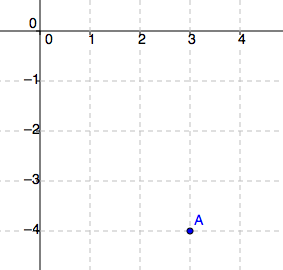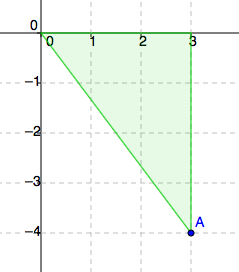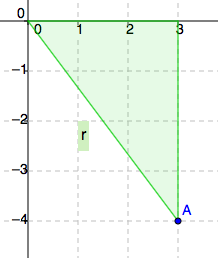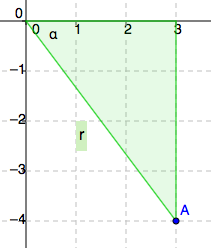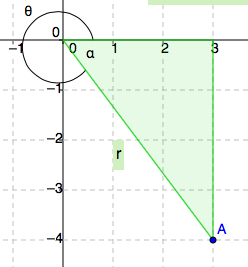### Home > PC > Chapter 11 > Lesson 11.2.1 > Problem11-70

11-70.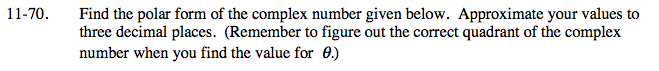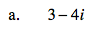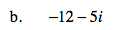Graph the point.

Draw a right triangle with the x-axis.

'r' is the length of the hypotenuse of the triangle. Calculate 'r' by using the Pythagorean theorem.

Use trigonometry to find the angle marked α.

Use the measure of angle α to find θ.